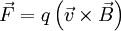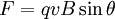# Magnetic field

(Redirected from Magnet)

A magnetic field is a vector field responsible for generating forces on electrically charged objects and magnets. Magnetic fields are generated by magnetic dipoles, moving electric charges, or changing electric fields.

Magnetic fields are related to electric fields; together they are part of electromagnetism. For instance, light is a propagating electric and magnetic wave. A magnetic force in one inertial frame of reference could correspond to an electric force, or to some combination of magnetic force and electric force, in another. This phenomenon is described by Maxwell's equations, and is a consequence of relativity.

## Mathematics

Magnetic fields are often denoted using the letter B. The magnetic force acting on a particle travelling at a velocity v can be written as:where q is the electrical charge on the particle. The cross product means that the force will always act at a right angle to both the field and the velocity and if the velocity is parallel to the field, no force will act. As the force always acts perpendicular to the velocity, a charged particle in a uniform (constant) magnetic field will undergo circular motion. The magnitude of the force can also be written as:where θ is the angle between the velocity and magnetic field. The direction can then be found using the right hand rule.

### Force on a Current Carrying Wire

An electrical current is rate of flow of charge past a point. By considering a wire with length L, the equation IL=nqv can be derived, where I is the current flowing along that wire and n is the "number density" (the number of charge carriers per unit length). This means that the force on a straight current carrying wire in a uniform magnetic field can be found as:with θ now being the angle between the wire and the magnetic field.

### Magnetic Field Produced by a Current Carrying Wire

Any electrical current creates a magnetic field. The magnetic field around a straight wire circulates around the wire. Its strength at a point depends only on the perpendicular distance to the wire and the size of the current:where r is the distance to the wire and μ0 is the permeability of free space.

## Earth's Magnetic Field

The magnetic field of Earth is directional nearly north-to-south, although slightly askance, meaning that "magnetic north" is not the same as "true north," and a person who is orienteering must take into account this change of declination, although it is truly only marginally relevant, unless you are close to either pole. This field has been decaying, in the time period that measurements have been made, at a rate of about about 5% per century. This decay suggests that, at some point, the poles will invert. In fact, geological evidence indicates that the magnetic field has reversed many times in the Earth's history. While the fluctuations are not uniform, reversals seem to take place about every 200,000 years, though the last reversal was about 780,000 years ago. The strength of the earth's magnetic field is according to the strength of the sun's magnetic field.

### Creationist View

One group that makes use of the Bible as a resource for science suggests that the history of the Earth's magnetic field is as depicted here.Change in the Earth's magnetic field according to Humphreys' model

The model, created by Russell Humphreys, can be summarized as:

• Creation of the Earth's magnetic field when God created the Earth
• A decay in its strength until the time of the Great Flood
• A series of very rapid reversals during the Flood, perhaps once every one or two weeks
• Fluctuations continue for around two thousand years after the Flood
• The steady decay of the field resumes

This model suggests the magnetic field of the Earth must be less than 10,000 years old.

This analysis is not accepted by the scientific community.